# Openstreetmap data – for Florence

Not that advanced, but I wanted to play around a bit with plotting the raw data from Openstreetmap.

We’re going to Florence this fall. It’s been five years since we last visited the fair city, that has played such an important role in western history.

Openstreetmaps is, as the name implies, open.

I’m going to need some libraries

``````#library(OpenStreetMap)
library(osmar)
library(ggplot2)
library(broom)
library(geosphere)
library(dplyr)
``````

osmar provides functions to interact with Openstreetmap. ggplot2 is used for the plots, broom for making some objects tidy and dplyr for manipulating data.

Getting the raw data, requires me to define a boundary box, encompassing the part of Florence I would like to work with. Looking at https://www.openstreetmap.org/export#map=13/43.7715/11.2717, I choose these coordinates:

``````top <- 43.7770
bottom <- 43.7642
left <- 11.2443
right <- 11.2661
``````

After that, I can define the bounding box, tell the osmar functions at what URL we can find the relevant API (this is just the default). And then I can retrieve the data via get_osm(). I immediately save it to disc. This takes some time to download, and there is no reason to do that more than once.

``````box <- corner_bbox(left, bottom, right, top)
src <- osmsource_api(url = "https://api.openstreetmap.org/api/0.6/")
florence <- get_osm(box, source=src)
saveRDS(florence, "florence.rda")
``````

Lets begin by making a quick plot:

``````plot(florence, xlim=c(left,right),ylim=c(bottom,top) )
``````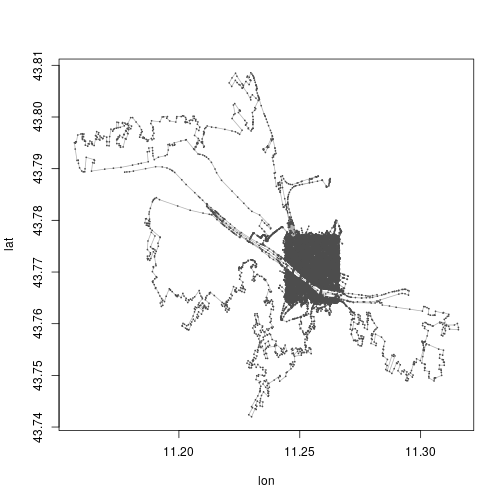Note that what we get a plot of, is, among other things, of all lines that are partly in the box. If a line extends beyond the box, we get it as well.

Looking at the data:

``````summary(florence\$ways)
``````
```## osmar\$ways object
## 6707 ways, 9689 tags, 59052 refs
##
## ..\$attrs data.frame:
##     id, visible, timestamp, version, changeset, user, uid
## ..\$tags data.frame:
##     id, k, v
## ..\$refs data.frame:
##     id, ref
##
## Key-Value contingency table:
##         Key         Value Freq
## 1  building           yes 4157
## 2    oneway           yes  456
## 3   highway    pedestrian  335
## 4   highway   residential  317
## 5   bicycle           yes  316
## 6       psv           yes  122
## 7   highway  unclassified  108
## 8   highway       footway  101
## 9   barrier          wall   98
## 10  surface paving_stones   87
```

I would like to plot the roads and buildings. For some reason there are a lot of highways, of a kind I would probably not call highways.

Anyway, lets make a list of tags. tags() finds the elements that have a key in the tag_list, way finds the lines that are represented by these elements, and find, finds the ID of the objects in “florence” matching this.
find_down() finds all the elements related to these id’s. And finally we take the subset of the large florence data-set, which have id’s matching the id’s we have in from before.

``````tag_list <- c("highway", "bicycle", "oneway", "building")
dat <- find(florence, way(tags(k %in% tag_list)))
dat <- find_down(florence, way(dat))
dat <- subset(florence, ids = dat)
``````

Now, in a couple of lines, I’m gonna tidy the data. That removes the information of the type of line. As I would like to be able to color highways differently from buildings, I need to keep the information.
Saving the key-part of the tags, and the id:

``````types <- data.frame(dat\$ways\$tags\$k, dat\$ways\$tags\$id)
names(types) <- c("type", "id")
``````

This gives me all the key-parts of all the tags. And I’m only interested in a subset of them:

``````types <- types %>%
filter(type %in% tag_list)

types\$id <- as.character(types\$id)
``````

Next as_sp() converts the osmar object to a spatial object (just taking the lines):

``````dat <- as_sp(dat, "lines")
``````

tidy (from the library broom), converts it to a tidy tibble

``````dat <- tidy(dat)
``````

That tibble is missing the types – those are added.

``````new_df <- left_join(dat, types, by="id")
``````

And now we can plot:

``````new_df %>%
ggplot(aes(x=long, y=lat, group=group)) +
geom_path(aes(color=type)) +
scale_color_brewer() +
xlim(left,right) +
ylim(bottom,top) +
theme_void() +
theme(legend.position="none")
``````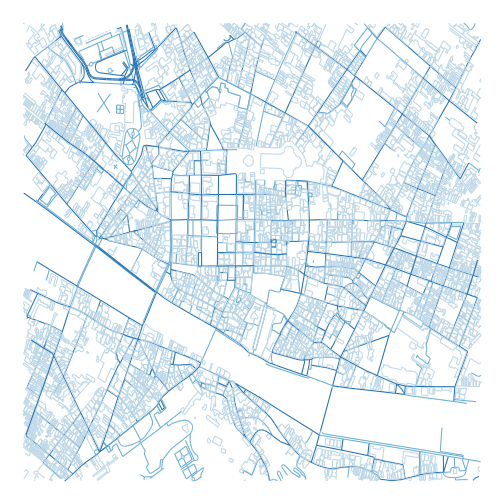Nice.

# Euler problem 62

The cube, 41063625 (3453), can be permuted to produce two other cubes: 56623104 (3843) and 66430125 (4053). In fact, 41063625 is the smallest cube which has exactly three permutations of its digits which are also cube.

Find the smallest cube for which exactly five permutations of its digits are cube.

Alright. I need to find five cubes, that are permutations of the same digits.

How to check if two numbers are permutations of each other?

We can generate the largest permutation of a given number. If the largest permutation of two numbers are identical, the two numbers are permutations of each other.

So I need a function, that returns the largest permutation of a number. It would be nice, if that function was vectorized.

``````max_perm <- function(t){
require(magrittr)
options(scipen=5)
t %>%
as.character() %>%
str_split("") %>%
lapply(sort, decreasing=TRUE) %>%
lapply(paste0, collapse="") %>%
unlist() %>%
as.double()
}
``````

Convert the input to character. Split at “”. That returns a list with vectors containing the individual digits of the input. lapply sorts the individual vectors in the list in decreasing order. Then lapply pastes the elements in each vector together with paste0 and “” as the separator. Then it is unlisted, and returned as numeric.

What is worth noting is a thing I was struggling with for far too long. R likes to write numbers in scientific notation. As in “1e+06”. I have not studied the phenomenon in detail. But options(scipen=5) solves the problem. It is the “penalty” used to decide when a number should be written in scientific notation. Unless I change that (trial and error, but it should be larger than whatever is default), as.character(1000000) will return “1e+06”. And the permutations of “1” “e” “+” “0” “6” are not terribly useful in this context.

I’m hazarding a guess that I don’t need to handle cubes of values of more than four digits.

Beginning with a vector of all numbers from 1 to 9999, I convert it to a dataframe. I transmute the first column to a column with the name x.
Then I mutate a second column, cube, into existence, and calculate it as the cube of the x-value. A third column, max_cube, is mutated with the result from my max_perm function above. And tha column is immediately used to group the data, so I get date grouped by identical maximum values of the permutations. I filter on the count of those groups, and only keep the groups that contain 5 elements. Then I ungroup it, and select just the cube column.

I now have a data frame with a single column containing 10 values. They are all cubes, five of them are permutations of each other. The other five are also permutaions of each other. And now I just have to take the smallest of them.

``````result <- 1:9999 %>%
as.double() %>%
as.data.frame() %>%
transmute(., x = .) %>%
mutate(cube = x**3) %>%
mutate(max_cube = max_perm(cube)) %>%
group_by(max_cube) %>%
filter(n()==5) %>%
ungroup() %>%
select(cube) %>%
min()
``````

Before I print the result, so I can enter it into Project Euler, I set options(digits=17).

Done! A good exercise. And a valuable lesson in the importance of the options in R.

# Crimes against graphs

Crime is a bad thing. No doubt about it. And one of the main topics in todays debate climate is – “those ‘orrible immigrants are very criminal. Look at these numbers, they prove it!”. Usually written with caps-lock engaged.

Well. Maybe they are, and maybe they do. But if you want to use statistics to prove it – pretty please, do not obfuscate the numbers.

This is an example. A blog post from one of the more notable danish newspapers. In the US it would be regarded as communist, in the rest of the world we would think of it as relatively conservative.

https://kulturkamp.blogs.berlingske.dk/2018/08/17/anmeldte-voldtaegter-og-voldsforbrydelser-er-paa-det-hoejeste-nogensinde/

The claim is, that the number of reported rapes and other violent crimes in Denmark, are the highest ever. That is because of the increasing numbers of immigrants in Denmark, especially muslims. Use Google translate if you want the details.

Again, that claim might be true. But the graphs in the post, that supposedly documents the claim, are misleading. To say the least.

First – the numbers come from the Danish Statistical Bureau. They have a disclaimer, telling us that changes to the danish penal code, means that a number of sexual offenses have been reclassified as violent crimes since 2013. If the number of violent crimes suddenly includes crimes that did not use to be classified as violent crimes, that number will increase. Not much of a surprise. Yes, the post asks why the numbers are still increasing after that reclassification. One should expect them to level off. And again the post may have a valid point. I don’t know. But what I do know, is that the graphs are misleading.

Heres why. The y-axis has been cut of. Lets recreate the graphs, and take a look.

There are two graphs. The first shows the number of reported cases of rape from 1995 until today.

The second shows the total number of reported cases of violent crimes in the same period. Both sets of data comes from http://www.statistikbanken.dk/.

We’re going to need some libraries:

``````library(ggplot2)
library(gridExtra)
``````

Lets begin by pulling the data.

There might be better ways, but I’ve simply downloaded the data. Two files:

``````violence <- read.csv("tab1.csv", sep=";", skip=3, header=F)
violence <- violence[1:(nrow(violence)-7),]
rape <- rape[1:(nrow(rape)-7),]
``````

The last seven lines are the notes about changes in which cases are counted in this statistics. I think that is a pretty important point, but they are difficult to plot.

The graph for rape, as presented in the post, and with a more sensible y-axis:

``````post <- ggplot(rape, aes(x=V1, y=V2)) +
geom_line(group=1) +
scale_x_discrete(breaks = rape\$V1[seq(1, length(rape\$V1), by = 20)]) +
theme_classic()

nice <- post + ylim(0,max(rape\$V2))
grid.arrange(post, nice, ncol=2)
``````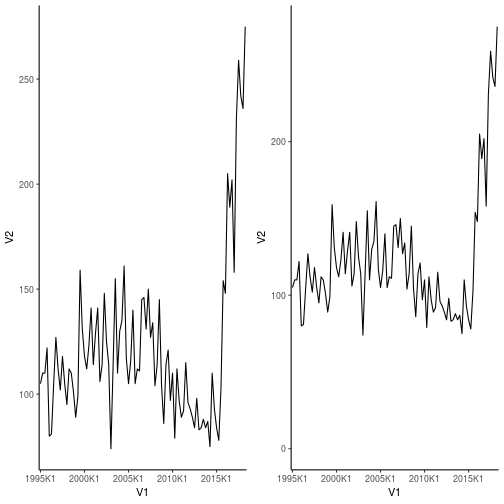And the one for violent crimes in general, again with the original on the left, and the better on the right:

``````post <- ggplot(violence, aes(x=V1, y=V2)) +
geom_line(group=1) +
scale_x_discrete(breaks = violence\$V1[seq(1, length(violence\$V1), by = 20)]) +
theme_classic()

nice <- post + ylim(0,max(violence\$V2))
grid.arrange(post, nice, ncol=2)
``````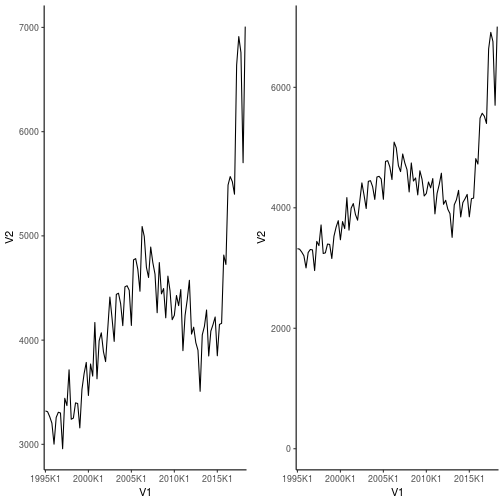So, still, some pretty scary increases. And the change in what is counted should give an increase. But that increase should level off, which it does not. Clearly something is not as it should be. But lets be honest, the graphs on the right are not quite as scary as the ones on the left.

Also – that change in what is counted as sexual assaults – it can explain the initial increase, but then it should level off. That is a fair point. However, there were other things that changed in the period. #metoo for example. I think it would be reasonable to expect that a lot of cases that used to be brushed of as not very important, are now finally being reported. The numbers might actually have leveled off without #metoo.

Anyway, my point is, that if you want to use graphs to support your claims, do NOT cut off the y-axis to make them look more convincing.

# Briefly unavailable for scheduled maintenance.

Oh the horror.

A couple of days ago something happened while updating one of my sites. “Briefly unavailable for scheduled maintenance.” was all that the page told me.

How to fix that? Noting that the page in question is made with WordPress?

Log on to the server serving your page. Delete the file “.maintenance”. Log out. Refresh.

If that doesn’t help, you have bigger problems.

Why is it happening? When you update something on a wordpress site, the site is placed in maintenance mode. It’s a bad idea to have people entering comments into the database at the same time you’re doing maintenance on it.

The site is placed in maintenance mode – every request for the site is given the messange “Briefly unavailable for scheduled maintenance”. And that is controlled by the file “.maintenance”. Normally that file will be deleted when the update or whatever is over. If something goes wrong, it survives. Everything went fine, but the file is still there, and your site is unavailable.

# The Mann-Whitney U test,

also known as the Mann-Whitney-Wilcoxon test, is a non-parametric test of a null hypothesis, that it is equally likely, that a randomly selected value from one sample, will be less than, or greater, than a randomly selected value from a second sample.

Intuitively I think I might be able to see how this translates to “the two samples are from populations that are actually different”. I have a harder time to describe that intuition.

The test is nearly as efficient as a t-test. But the advantadge is, that it does not require that we can assume that the two samples are normal distributed.

It can also be used to test if two independent samples, were selected from populations that have the same distribution.

The idea is, we have to samples. If we pick a random value from sample A, and a random value from sample B, there is a likelihood, a chance, that the value from sample A is larger than the value from sample B. Is that likelihood the same as the likelihood that the value from sample A is smaller than the value from sample B.

# How to do that in R?

Let us look at some data. mtcars is a dataset with some facts about various 1974 US cars.
One of the numbers we have i mpg, miles pr gallon, or fuel-efficiency. Another is about the transmission. Is there a manual or an automatic gearbox. In the column am a “1” indicates an automatic gearbox. A “0” a manual gearbox.

We can now extract two sets of data on the fuel efficiency, based on the type of transmission:

``````aut <- mtcars[which(mtcars\$am==1),]\$mpg
man <- mtcars[which(mtcars\$am==0),]\$mpg
``````

In “aut” we have the fuel efficiency of cars with an automatic gearbox, and in “man” the same, but for the, in Europe, more familiar, manual gearbox.

How to do the Wilcoxon test?

``````wilcox.test(aut,man)
``````
```## Warning in wilcox.test.default(aut, man): cannot compute exact p-value with
## ties
```
```##
##  Wilcoxon rank sum test with continuity correction
##
## data:  aut and man
## W = 205, p-value = 0.001871
## alternative hypothesis: true location shift is not equal to 0
```

The p-value is 0.001871. If we take a 0.05 significance level, we can conclude that the two sets of values are from non-identical populations. Had it been larger (quite a bit larger), we would not have been able to reject the null-hypothesis, that the two samples comes from identical populations.

There is a different way to do the test, where we dont split the dataset in two:

``````wilcox.test(mpg ~ am, data=mtcars)
``````
```## Warning in wilcox.test.default(x = c(21.4, 18.7, 18.1, 14.3, 24.4, 22.8, :
## cannot compute exact p-value with ties
```
```##
##  Wilcoxon rank sum test with continuity correction
##
## data:  mpg by am
## W = 42, p-value = 0.001871
## alternative hypothesis: true location shift is not equal to 0
```

We tell the function that we want to test mpg (fuel efficiency), as a function of am (the type of transmission), and that the dataset we are working on is mtcars. The function then splits the dataset in two based on the value in am. Had there been more than two, things would probably stop working.

The result is the same though.

# The warning

We get a warning. That is because the algorithm has a problem when there are identical values in the two datasets. There is a way around. We can add a “jitter”, to the two datasets. jitter() adds small random values to the values in the dataset. Often that solves the problem:

``````wilcox.test(jitter(aut),jitter(man))
``````
```##
##  Wilcoxon rank sum test
##
## data:  jitter(aut) and jitter(man)
## W = 205, p-value = 0.001214
## alternative hypothesis: true location shift is not equal to 0
```

On the other hand, we are no longer comparing the values in the dataset. We can see the difference here:

``````aut - jitter(aut)
``````
```##   -0.06068697  0.06873662  0.05276257  0.06029616 -0.04756118
##   -0.03489005 -0.01479096 -0.04547827 -0.06275216  0.01547043
##  -0.02540212 -0.05201828  0.01065800
```

It would probably be a bad idea to add random noise to the data. On the other hand, it is not very likely that two cars have exactly the same fuel efficiency. We have probably “binned” the values, and the addition of random noise would probably not change the values we are working on too much. But you should always consider why the warning arises, if it is actually a problem and not just a warning, and if it is appropriate to solve it in this way.

# dplyr::count()

A short note for myself.

Given a dataframe, eg mtcars:

``````head(mtcars)
``````
```##                    mpg cyl disp  hp drat    wt  qsec vs am gear carb
## Mazda RX4         21.0   6  160 110 3.90 2.620 16.46  0  1    4    4
## Mazda RX4 Wag     21.0   6  160 110 3.90 2.875 17.02  0  1    4    4
## Datsun 710        22.8   4  108  93 3.85 2.320 18.61  1  1    4    1
## Hornet 4 Drive    21.4   6  258 110 3.08 3.215 19.44  1  0    3    1
## Hornet Sportabout 18.7   8  360 175 3.15 3.440 17.02  0  0    3    2
## Valiant           18.1   6  225 105 2.76 3.460 20.22  1  0    3    1
```

These cars have 4, 6 or 8 cylinders. How many cars fall in each category?

``````count(mtcars, cyl)
``````
```## # A tibble: 3 x 2
##     cyl     n
##   <dbl> <int>
## 1    4.    11
## 2    6.     7
## 3    8.    14
```

They have 3, 4 or 5 gears.

How many cars have 8 cylinders, and 3 gears?

``````count(mtcars, cyl, gear)
``````
```## # A tibble: 8 x 3
##     cyl  gear     n
##   <dbl> <dbl> <int>
## 1    4.    3.     1
## 2    4.    4.     8
## 3    4.    5.     2
## 4    6.    3.     2
## 5    6.    4.     4
## 6    6.    5.     1
## 7    8.    3.    12
## 8    8.    5.     2
```

This is equivalent to first grouping the observations by cylinders and gears. And then counting using tally():

``````mtcars %>%
group_by(cyl,gear) %>%
tally()
``````
```## # A tibble: 8 x 3
## # Groups:   cyl [?]
##     cyl  gear     n
##   <dbl> <dbl> <int>
## 1    4.    3.     1
## 2    4.    4.     8
## 3    4.    5.     2
## 4    6.    3.     2
## 5    6.    4.     4
## 6    6.    5.     1
## 7    8.    3.    12
## 8    8.    5.     2
```

If, for some obscure reason, we want to count the number of carburetors in each category, we can do that:

``````count(mtcars, cyl, gear, wt=carb)
``````
```## # A tibble: 8 x 3
##     cyl  gear     n
##   <dbl> <dbl> <dbl>
## 1    4.    3.    1.
## 2    4.    4.   12.
## 3    4.    5.    4.
## 4    6.    3.    2.
## 5    6.    4.   16.
## 6    6.    5.    6.
## 7    8.    3.   37.
## 8    8.    5.   12.
```

The cars with 8 cylinders, and 3 gears, have among them a total of 37 carburetors.

# Euler 42

A triangle number is given by t~n~ = ½(n(n+1))

Lets start by writing a function for that:

``````triangle <- function(n){
(n*(n+1))/2
}
``````

If we take a word, Project Euler uses SKY as an example, we can take each letter, assign to it the value of its position in the alphabet, and add together the values of the letters.
SKY becomes 19 + 11 + 25 = 55.

Lets write a function that finds that:

``````lettervalue <- function(chars){
chars <- toupper(chars)
t <- unlist(strsplit(chars,""))
sum(match(t, LETTERS))
}
``````

First I convert the character string in the input to uppercase letters. This might not be necessary.
Then I split the string in individual characters. And unlist() the result.
Then I pass it to the match() function. That gives me the position of a character as a numerical value, in the vector LETTERS.
Neat function.
Then I sum it.

This is nice and vectorized all the way through.

But what was the problem I should solve?
Project Euler provides a list of words. How many of these are, when converted to a sum with the function above, a triangle number?

I have no idea about how long a given word is. But lets find out.

First, lets read in the file.

``````wordlist <- scan("p042_words.txt", what=character(), sep=",", quiet=TRUE)
``````

What are the numerical values as defined above?
My function lettervalue just adds everything together. Which is a bit unhelpful. If I use map() from the purrr-libray, I can apply the function to each element in wordlist. I’ll have to unlist() it afterwards. That gives me all the numerical values:

``````library(purrr)
values <- unlist(map(wordlist, lettervalue))
``````

What is the largest of them?

``````max(values)
``````
```##  192
```

That is the highest number I have to check is triangular.

Lets generate a list of the first 20 triangle numbers:

``````t <- 1:20
triangles <- triangle(t)
max(triangles)
``````
```##  210
```

That was lucky, triangle-number 20 is 210, which is larger than the largest value I have in the set of words.
Now I just need to find out how many of the values in values are in the variable triangles:

``````answer <- sum(values%in%triangles)
``````

Neat.
Lessons learned:
1. map() can be used to apply a function to a vector. Even functions that are not that well behaved.
And it’s pretty damn fast. An alternative would be one of the apply-functions:

``````library(microbenchmark)
map_test <- function(){map(wordlist, lettervalue)}
apply_test <- function() {lapply(wordlist, lettervalue)}
mbm <- microbenchmark(map_test, apply_test, times=1000)
library(ggplot2)
autoplot(mbm)
``````
```## Warning: Transformation introduced infinite values in continuous y-axis
```
```## Warning: Removed 915 rows containing non-finite values (stat_ydensity).
```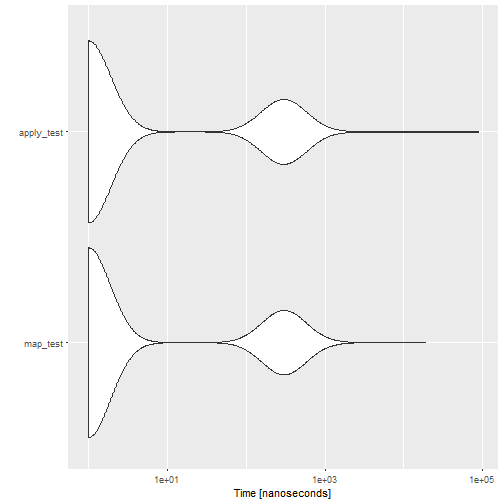1. match() is also pretty useful. I spend a couple of frustrated minutes trying to get a list to behave as a lookup-table. match() is much easier.

# Euler 2

The second Project Euler problem.

Given the Fibonacci sequence, calculate all terms below 4 million. What is the sum of the even terms?

We are going to use the library “numbers” a lot!
It includes a function, that allows us to generate the Fibonacci-sequence. But how many terms should we get?
By inspection we find that the 34th term is:

``````library(numbers)
fibonacci(34)
``````
```##  5702887
```

Therefore we only need the 33 first terms:

``````(f <- fibonacci(33, sequence = TRUE))
``````
```##         1       1       2       3       5       8      13      21
##        34      55      89     144     233     377     610     987
##     1597    2584    4181    6765   10946   17711   28657   46368
##    75025  121393  196418  317811  514229  832040 1346269 2178309
##  3524578
```

We only have to sum the even terms:

``````sum(f[!f%%2])
``````
```##  4613732
```

Lets split that up a bit. %% i modulus. It returns what is “left” in the division.
4%%2 returns 0, because there is no remainder – because 4 is an equal number.
When we do that on all the terms in the sequence, we get:

``````f%%2
``````
```##   1 1 0 1 1 0 1 1 0 1 1 0 1 1 0 1 1 0 1 1 0 1 1 0 1 1 0 1 1 0 1 1 0
```

0 for the equal terms, 1 for the unequal terms.
0 is equivalent to FALSE, and 1 to TRUE.
And ! negates the logical value:

``````!f%%2
``````
```##   FALSE FALSE  TRUE FALSE FALSE  TRUE FALSE FALSE  TRUE FALSE FALSE
##   TRUE FALSE FALSE  TRUE FALSE FALSE  TRUE FALSE FALSE  TRUE FALSE
##  FALSE  TRUE FALSE FALSE  TRUE FALSE FALSE  TRUE FALSE FALSE  TRUE
```

Compare that with the original sequence – now the value is FALSE for the unequal numbers, and true for the equal.
We can use that to pick out the equal terms in the sequence:

``````f[!f%%2]
``````
```##         2       8      34     144     610    2584   10946   46368
##    196418  832040 3524578
```

And then it is simple enough to just sum them:

``````sum(f[!f%%2])
``````
```##  Censored
```

Lessons learned:
1. You should always save your Euler solutions, otherwise you will have to reconstruct them when you decide to put them all on your website three years later.
2. Numbers is a really usefull library.

# Merging PDF-files

You may need to merge several pdf-files into one.

There are several tools. Some of them you have to pay for.

If you are in a linux environment, try this:

gs -dBATCH -dNOPAUSE -q -sDEVICE=pdfwrite -sOutputFile=final.pdf filea.pdf fileb.pdf

continue with filec.pdf etc.

Some say it is slow.

Others say, yes it is slow, but it produces smaller final files, and are able to handle “difficult pdfs”.

I say it is nice that it can be done from the command line.